# COLLIGATIVE PROPERTIES STATE PERFORMANCE OBJECTIVES Calculate concentration in

• Slides: 19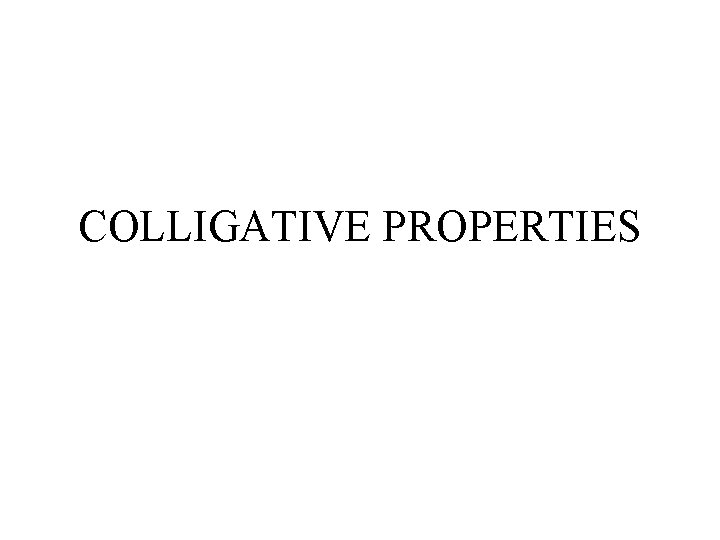COLLIGATIVE PROPERTIES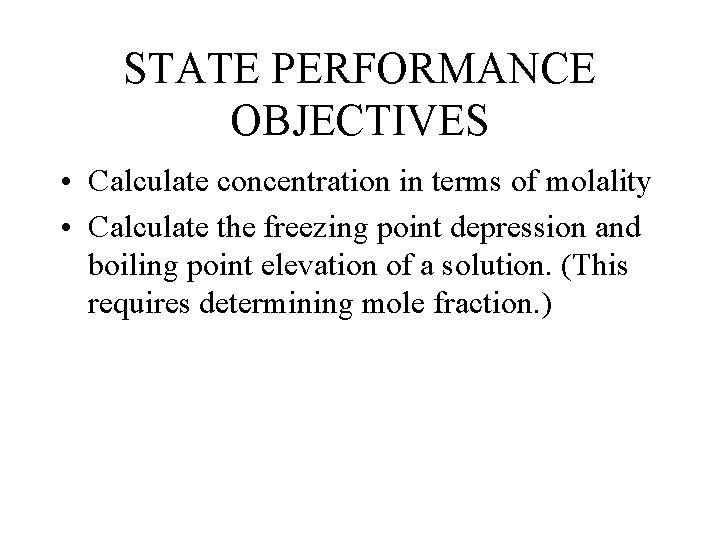STATE PERFORMANCE OBJECTIVES • Calculate concentration in terms of molality • Calculate the freezing point depression and boiling point elevation of a solution. (This requires determining mole fraction. )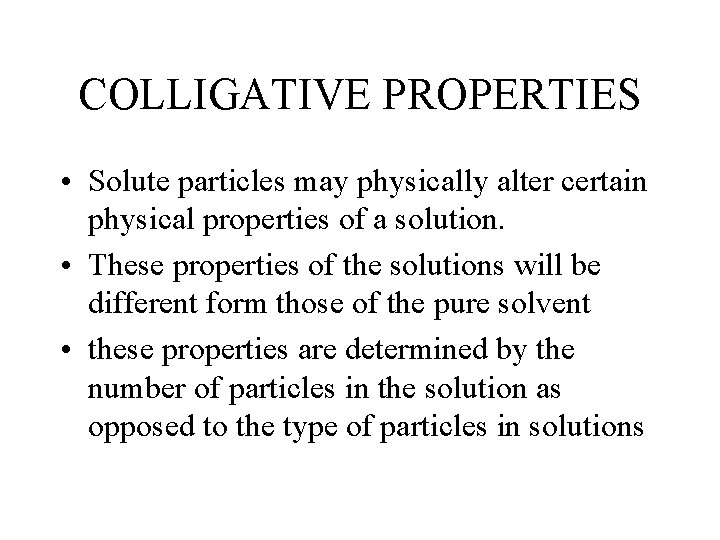COLLIGATIVE PROPERTIES • Solute particles may physically alter certain physical properties of a solution. • These properties of the solutions will be different form those of the pure solvent • these properties are determined by the number of particles in the solution as opposed to the type of particles in solutions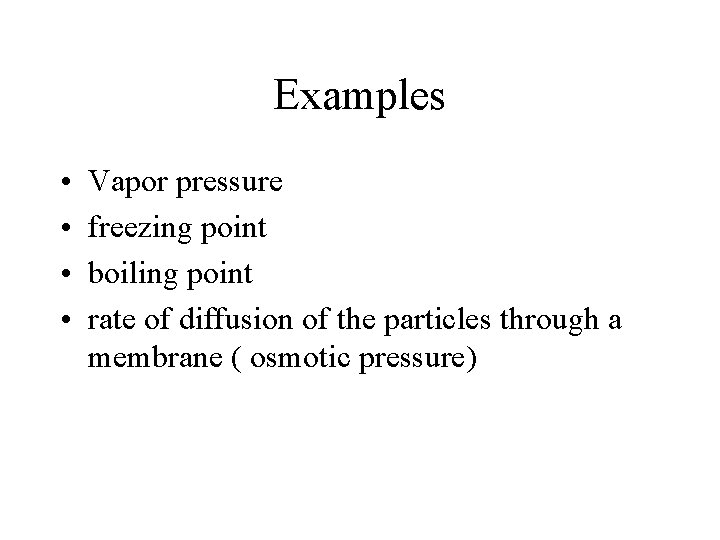Examples • • Vapor pressure freezing point boiling point rate of diffusion of the particles through a membrane ( osmotic pressure)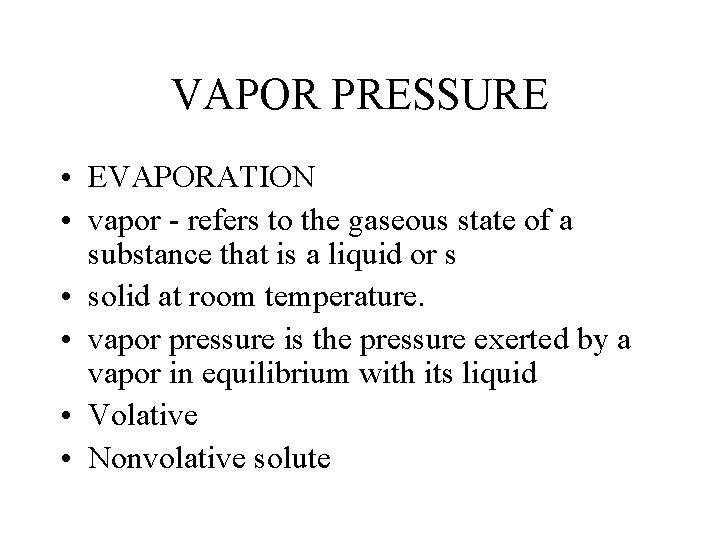VAPOR PRESSURE • EVAPORATION • vapor - refers to the gaseous state of a substance that is a liquid or s • solid at room temperature. • vapor pressure is the pressure exerted by a vapor in equilibrium with its liquid • Volative • Nonvolative soluteVAPOR PRESSURE • A solution that contains a nonvolative solute always has a lower VP than does the pure solvent • Dec in VP is directly related to the # of particles of solute • If substance dissociates – it has greater impact on VP than 1 that does not dissociates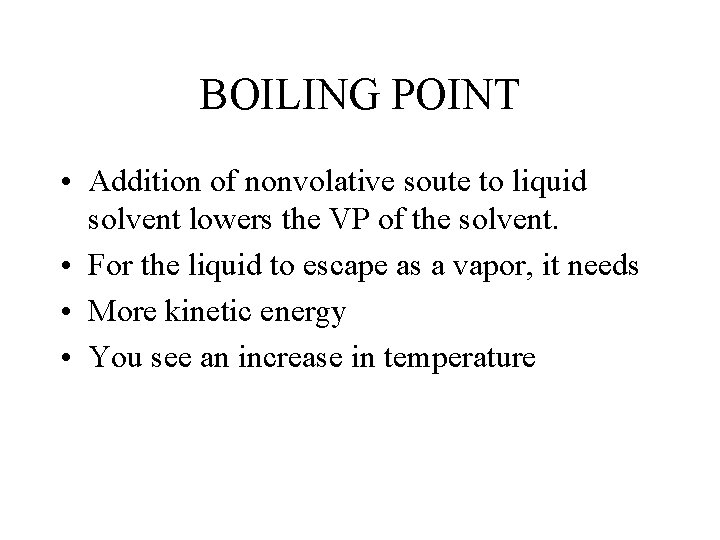BOILING POINT • Addition of nonvolative soute to liquid solvent lowers the VP of the solvent. • For the liquid to escape as a vapor, it needs • More kinetic energy • You see an increase in temperatureFREEZING POINT • When a substance freezes, the particles take on an orderly patter • Solutes disrupts this formation. • More kinetic energy must be removed from the solution in order for the solvent to solidify. The freezing point is lowered.MOLALITY • The property of the solution is dependent upon the number of solute particles present within a given mass of solvent. • This makes it more convenient to express the concentration of the solution in terms of moles of solution per mass of solvent • formula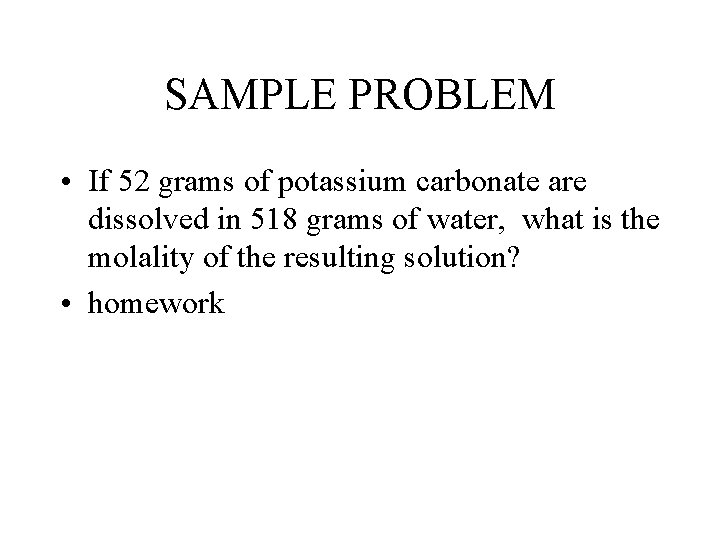SAMPLE PROBLEM • If 52 grams of potassium carbonate are dissolved in 518 grams of water, what is the molality of the resulting solution? • homeworkRAOULTS’ LAW • Any nonvolative solute at a specific concentration lowers the vapor pressure of a solvent by an amount that is characteristic of that solvent.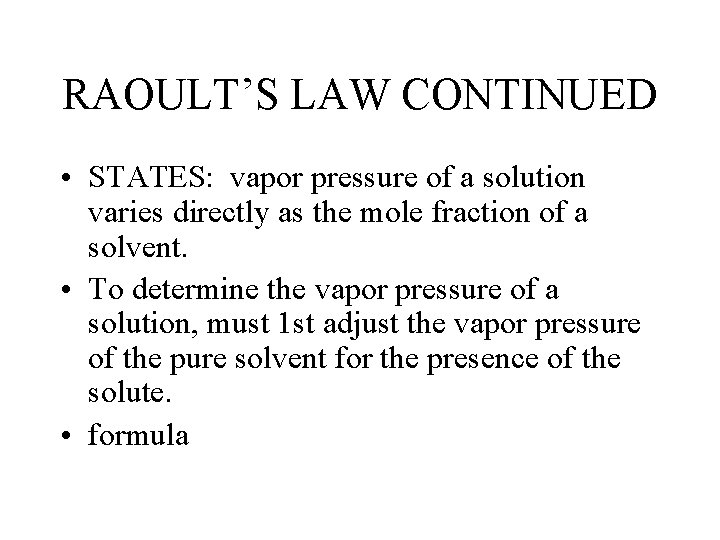RAOULT’S LAW CONTINUED • STATES: vapor pressure of a solution varies directly as the mole fraction of a solvent. • To determine the vapor pressure of a solution, must 1 st adjust the vapor pressure of the pure solvent for the presence of the solute. • formulaSAMPLE PROBLEM • Sugar is extremely soluble in water and is not a volatile substance. What would the vapor pressure of water be at 700 C if 1. 0 x 102 grams of water have dissolved 2 x 102 grams of sucrose?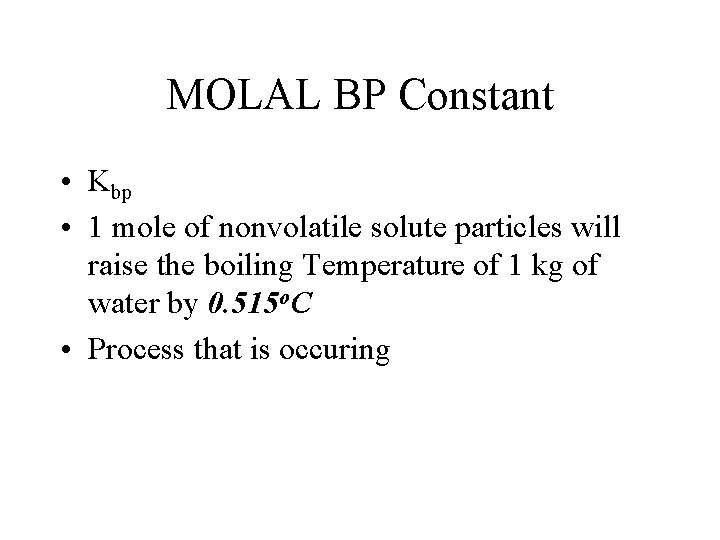MOLAL BP Constant • Kbp • 1 mole of nonvolatile solute particles will raise the boiling Temperature of 1 kg of water by 0. 515 o. C • Process that is occuring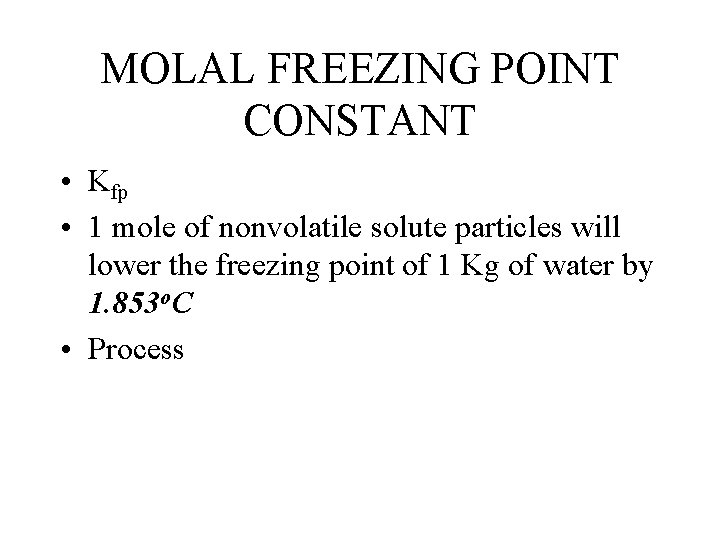MOLAL FREEZING POINT CONSTANT • Kfp • 1 mole of nonvolatile solute particles will lower the freezing point of 1 Kg of water by 1. 853 o. C • Process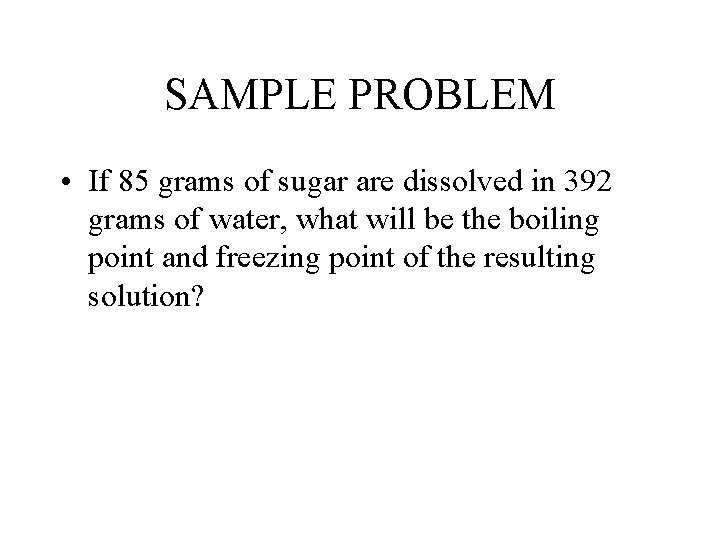SAMPLE PROBLEM • If 85 grams of sugar are dissolved in 392 grams of water, what will be the boiling point and freezing point of the resulting solution?IONIC SOLUTIONS • Will affect the BP and FP of the solutions • Must adjust for the moles of the ionic compound because of dissociationSAMPLE PROBLEM • If 26. 4 grams of nickel(II)bromide are dissolved in 224 grams of water, what will be the boiling and freezing point of the resulting solution. Assume 100% ionization of the compound.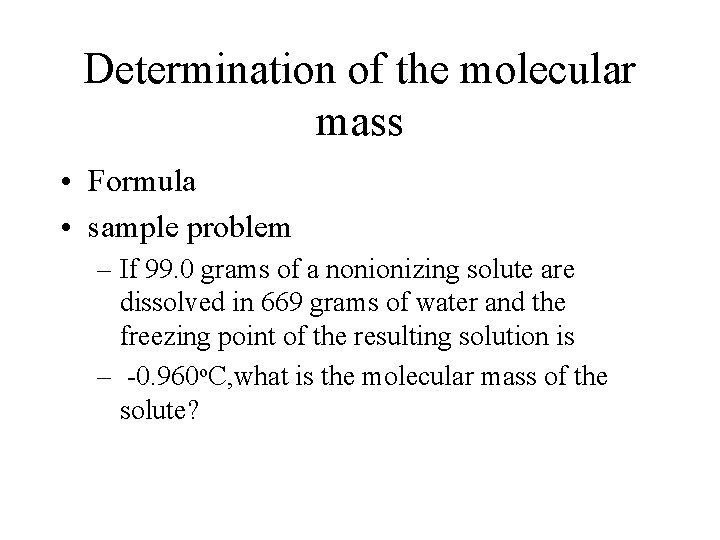Determination of the molecular mass • Formula • sample problem – If 99. 0 grams of a nonionizing solute are dissolved in 669 grams of water and the freezing point of the resulting solution is – -0. 960 o. C, what is the molecular mass of the solute?